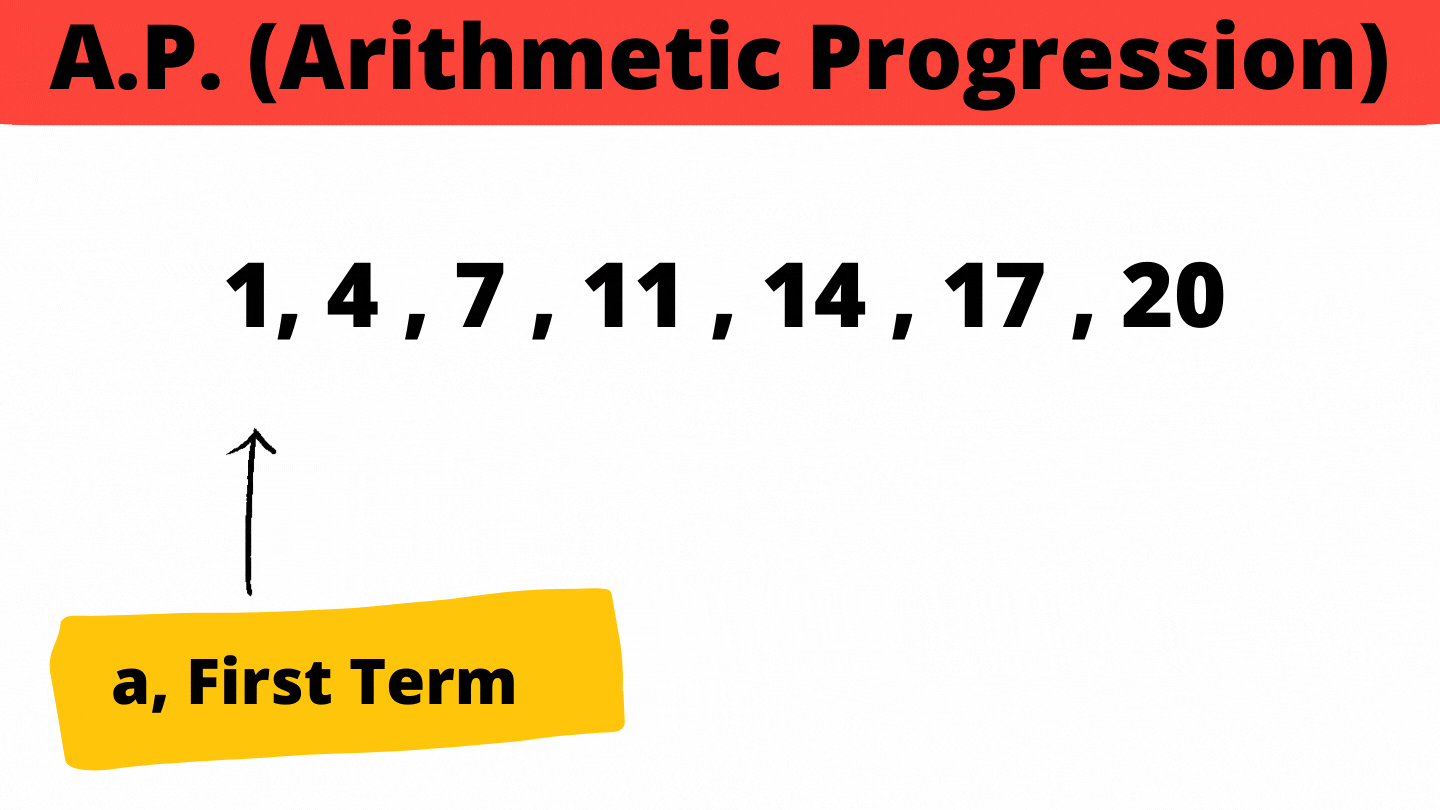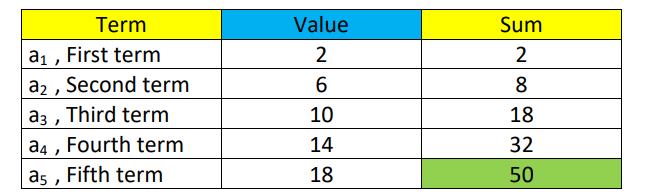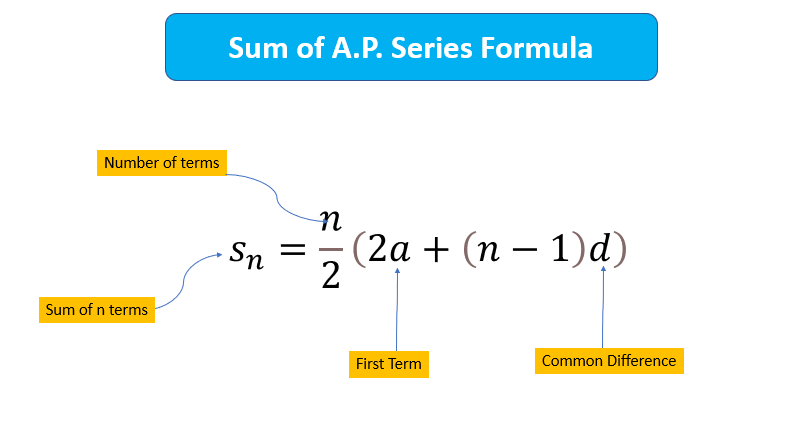# Find Sum of AP Series

Problem Statement: Given an A.P. Series, we need to find the sum of the Series.

a = first term of A.P.

d= common Difference of A.P.

n= Number of Terms in  A.P.

Examples:

```Example 1:
Input:
n=4
a=2
d=2
Output: 20
Explanation: 2+4+6+8 = 20

Input:
n=8
a=2
d=5
Output: 124
Explanation: -2 +3 + 8 + 13 + 18 + 23 + 28 + 33 = 124```

What is A.P. (Arithmetic Progression)?

A.P. is the series of terms having the first term as a and d, common difference. Every next term in the A.P. is greater than the previous term by d units.

Example –

-2, 3 , 8 , 13 , 18 , 23 , 28 , 33

First term , a = – 2

Common Difference , d=5

Last term , an=33### Solution

DisclaimerDon’t jump directly to the solution, try it out yourself first.

Solution 1: Using Loops

Approach: This approach consists of simply adding elements by moving them for a loop. We create a sum variable and add the terms one by one to get the sum of the A.P. Series.

For Example,

a=2 , d=4, n=5

So run the loop 5 times.Code:

## C++ Code

``````#include <bits/stdc++.h>
using namespace std;
float sumofAp(float a, float d, int n)
{
float sum = 0;
for (int i = 1; i <= n; i++)
{
sum += a;
a += d;
}
return sum;
}
int main()
{
float a = 1.5, d = 3;
int n = 5;
cout << "Sum of Given Ap Series: " << sumofAp(a, d, n) << endl;
}
``````

Output:

Sum of Given Ap Series: 37.5

Time Complexity: O(n) where n is the number of terms in the A.P. Series

Space Complexity: O(1) since no extra space is required

## Java Code

``````import java.util.*;
class TUF{
static double sumofAp(double a, double d, int n)
{
double sum = 0.0;
for (int i = 1; i <= n; i++)
{
sum += a;
a += d;
}
return sum;
}
public static void main(String args[])
{
double a = 1.5, d = 3;
int n = 5;
System.out.println("Sum of Given Ap Series: "+sumofAp(a, d, n));
}
}``````

Output:

Sum of Given Ap Series: 37.5

Time Complexity: O(n) where n is the number of terms in the A.P. Series

Space Complexity: O(1) since no extra space is required

Solution 2: Using Formula

Approach: This approach is now a time as well as a space-optimized approach since we are going to use the direct formula for this.Code:

``````#include <bits/stdc++.h>
using namespace std;
float sumofAp(float a, float d, int n)
{
float sum = (n / 2.0) * (2.0 * a + (n - 1) * d);
return sum;

}
int main()
{
float a = 1.5, d = 3, n = 5;
cout << "Sum of Given Ap Series: " << sumofAp(a, d, n) << endl;
}
``````

Output:

Sum of Given Ap Series: 37.5

Time Complexity: O(1) since we are using direct formula

Space Complexity: O(1) since no extra space is required

## Java Code

``````import java.util.*;
class TUF{
static double sumofAp(double a, double d, int n)
{
double sum = (n / 2.0) * (2.0 * a + (n - 1) * d);
return sum;
}
public static void main(String args[])
{
double a = 1.5, d = 3;
int n = 5;
System.out.println("Sum of Given Ap Series: "+sumofAp(a, d, n));
}
}``````

Output:

Sum of Given Ap Series: 37.5

Time Complexity: O(1) since we are using direct formula

Space Complexity: O(1) since no extra space is required

Special thanks to Gurmeet Singh for contributing to this article on takeUforward. If you also wish to share your knowledge with the takeUforward fam, please check out this article